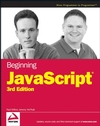Wrox Programmer ForumsChapter 6: Trivia Quiz
 |
BOOK: Beginning JavaScript 3rd Ed. ISBN: 978-0-470-05151-1This is the forum to discuss the Wrox book Beginning JavaScript, 3rd Edition by Paul Wilton, Jeremy McPeak; ISBN: 9780470051511 Read more about Beginning JavaScript, 3rd Edition or buy the book from your favorite retailerDownload the code for Beginning JavaScript, 3rd Edition
 Welcome to the p2p.wrox.com Forums. You are currently viewing the BOOK: Beginning JavaScript 3rd Ed. ISBN: 978-0-470-05151-1 section of the Wrox Programmer to Programmer discussions. This is a community of software programmers and website developers including Wrox book authors and readers. New member registration was closed in 2019. New posts were shut off and the site was archived into this static format as of October 1, 2020. If you require technical support for a Wrox book please contact http://hub.wiley.com

 zero_coolUKRegistered User Join Date: May 2009 Posts: 1 Thanks: 0 Thanked 0 Times in 0 PostsChapter 6: Trivia Quiz

I'm trying to adapt the code for the trivia quiz from chapter 6 for my own quiz on my website.

However, on my quiz I have all of my questions set up on one page, rather than picking one at random. The problem is, the code produces no errors but returns every question option as wrong no matter whether I select the option that I have declared as correct or not.

Here's my code:

Code:
```
{
// declare a variable to hold return value
var correct = false;

// if answer provided is same as correct answer then correct variable is true
correct = true;

// return whether the answer was correct (true or false)
return correct;
}

function buttonCheckQ_onclick()
{

{
}
answer = String.fromCharCode(65 + answer); // convert the radio button index found by the while loop into a character (uses character codes, 65 is A so if user chooses button 0 then 0+65 = A)

{
alert("You got it right"); // if the index number of the answer matches the one held in the array answers, display an alert informing the user
}
else
{
alert("You got it wrong"); // if the index number of the selected radio button does not match the one held in array answers, display a different alert
}
window.location.reload(); // refresh the page once the user has clicked Check Answer
}

var questions = new Array();

questions = new Array; // define question 1 as an array; as the questions are multiple choice so the array for the questions needs to be multi-dimensional

questions = "When was ESP founded?"; // text for question 1

questions = "1965"; // choices for answer set out as radio buttons on quiz form
questions = "1995";
questions = "1975";
questions = "1985";

questions = new Array;
// define text and answer for question 2
questions = "Which US city did ESP move to from Japan?";

questions = "New York";
questions = "Los Angeles";
questions = "San Francisco";
questions = "Houston";

questions = new Array;
// define question 3
questions = "And where did the company relocate to in 1993";

questions = "San Francisco";
questions = "Chicago";
questions = "Los Angeles";
questions = "Boston";

questions = new Array;
// define question 4
questions = "How many artists have had signature guitars made for them by ESP";

questions = "20";
questions = "21";
questions = "18";
questions = "24";

questions = new Array;
// define question 5
questions = "How long have I been playing guitar for?";

questions = "11 years";
questions = "7 years";
questions = "12 years";
questions = "10 years";

questions = new Array;
//define question 6
questions = "How many notes are there on a guitar with a standard-scale neck";

questions = "132";
questions = "122";
questions = "142";
questions = "112";

//-->
</script>```
As you can see, the code is identical to that from the book but I have removed questionNumber from the answerCorrect function as I want to check each question on the page one at a time, rather than having questions appear one at a time at random.

Any help on this will be much appreciated.